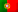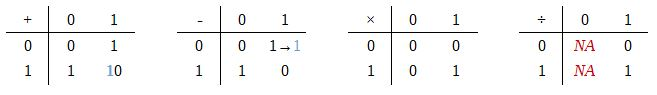Home > Technology > Computer Science > Computer Architecture > Arithmetic operations in binary

# Arithmetic operations in binary

In a previous post, we have seen that computers are limited to 2 states: ON/OFF; True/False; 1/0. But it is easy to explain! Basically, logical circuits that perform “AND”, “OR”, “Exclusive-OR” and “NOT” boolean operation are implemented all over the computer hardware. So, the given “True” or “False” results are easily represented by 1 or 0, respectively. That’s the main reason to use the binary system on computers.

In this post we will going to talk about arithmetic operations in binary.

It might sound strange to perform binary calculations, but in fact it is not that different from “normal” rules. To understand this, let’s take an example and compare the addition in decimal and the addition in binary:

181 + 51

If we go back to the primary school times…

 (1) 1 8 1 + 5 1 2 3 2

Let’s try to remember the steps: 1 plus 1 equals 2; 8 plus 5 equals 13 which is greater than the radix (10), so we have 3 and carry 1; finally the carried 1 plus 1 equals 2. Keep in mind the “carry 1” case… We will find it again!

Let’s now see the operation in binary with the equivalent values:

1 0 1 1 0 1 0 1 (2) + 1 1 0 0 1 1 (2)

 (1) (1) (1) (1) (1) 1 0 1 1 0 1 0 1 + 1 1 0 0 1 1 1 1 1 0 1 0 0 0

Let’s now follow the step-by-step sums since the LSB (Less Significant Bit, the rightmost bit) to the MSB (Most Significant Bit, the leftmost bit):

• 1 plus 1 equals “10”, 0 and carry 1

• 1 plus 0 plus 1 equals “10”, 0 and carry 1

• 1 plus 1 plus 0 equals “10”, 0 and carry 1

• 1 plus 0 plus 0 equals 1

• 1 plus 1 equals “10”, 0 and carry 1

• 1 plus 1 plus 1 equals “11”, 1 and carry 1

• 1 plus 0 (plus 0) equals 1

• 1

If you find strange to see “1 + 1 = 10” and “1 + 1 + 1 = 11”, remember we are working in binary where “10” equals 2 in decimal and “11” equals 3 in decimal…

The “1’s” around brackets represent the carried digits. When we go deep into the processor level, there is a bit flag called “Carry Flag” or “Carrier” which becomes 1 whenever “carry 1” case is found.

## Subtraction in binary

No special changes regarding to subtraction. We will see again the “carry 1” but in this case it will be used to subtract a minuend digit.

First, let’s take again an example in decimal:

172 – 85

 (1) (1) 1 (0) 7 (6) 2 - 8 5 0 8 7

Now we have: 2 minus 5 does not fit, so we have 12 minus 5 equals 7 and carry 1. To the minuend number 7 we subtract the carry, so we have 7 minus 1 equals 6 and “6 – 8″ (16 – 8) equals 8 and carry 1 again. Finally 1 minus 1 equals 0.

Now in binary, using the same numbers:

1010 1100 – 101 0101

 (1) (1) (1) (1) (1) 1 (0) 0 1 (0) 0 1 (0) 1 (0) 0 (1) 0 - 1 0 1 0 1 0 1 0 1 0 1 0 1 1 1

Let’s follow step-by-step the results we have:

• 0 – 1, carry 110 – 1 = 1 (remember that 10 (2) = 2 (10))

• Subtracts the carry 1 to the minuend digit: 01, which will carry 1 again and we’ll have 1 – 0 = 1

• Subtracts the carry 1: 1 – 1 = 0, and 0 – 1, carry 110 – 1 = 1

• Subtracts another carried digit: 1 – 1 = 0, and 0 – 0 = 0

• 0 – 1, carry 110 – 1 = 1

• Subtracts another carried digit: 1 – 1 = 0, and 0 – 0 = 0

• 0 – 1, carry 110 – 1 = 1

• Subtracts another carried digit: 1 – 1 = 0, and 0 – 0 = 0

Finally we get the binary number: 101 0111 which is 87 in decimal!

## Multiplication in binary

And, what about multiplying binaries? Would it be harder? Let’s see…

152 × 19

 1 5 2 x 1 9 1 3 6 8 + 1 5 2 2 8 8 8

Let’s now see in binary…

1001 1000 × 1 0011

 1 0 0 1 1 0 0 0 x 1 0 0 1 1 1 0 0 1 1 0 0 0 1 0 0 1 1 0 0 0 0 0 0 0 0 0 0 0 0 0 0 0 0 0 0 0 + 1 0 0 1 1 0 0 0 1 0 1 1 0 1 0 0 1 0 0 0

Extremely easy! Especially in this example where we had a lot of zeros, which helped performing the sums. And, in fact:

1011 0100 1000 (2) = 2888 (10)

If, however, the sum of the columns has a lot of ones it may be harder to perform the sums. So, it might be a good idea to perform some partial sums. For instance:

 1 0 1 1 1 1 x 1 1 0 1 1 0 1 1 1 1 + 0 0 0 0 0 0 0 1 0 1 1 1 1 + 1 0 1 1 1 1 1 1 1 0 1 0 1 1 + 1 0 1 1 1 1 0 0 1 0 0 1 1 0 0 0 1 1

Let’s check this out: 10 1111 (2) = 47 (10) ; 1101 (2) = 13 (10) ; 47 × 13 = 611 ; 10 0110 0011 (2) = 611 (10)

## Division in binary

Regarding division, we will need to think a little but it is not much different from decimal.

Let’s take the example 89 ÷ 7

 8'9 7 1 9 12 5

Let’s go back again to the primary school and let’s not use decimal places:

• 89 ÷ 7 : the tens is 8, so 8 ÷ 7 = 1, remainder 1
• Joining the uits, we get 19 ÷ 7 = 2, remainder 5
• So, the final integer result is 12 and the remainder is 5

Let’s check this values in binary: 101 1001 (2) ÷ 111 (2) and let’s use partial subtractions to help performing the calculations:

 1 0 1 1'0 0 1 1 1 1 - 1 1 1 1 1 0 0 0 1 0 0 1 - 1 1 1 0 0 0 1 0 - 0 0 0 0 1 0 1 - 0 0 0 1 0 1

So, step-by-step we have:

• We need to start with 1011 so we can divide it by 111
• We write a 1 in the quocient and get 111 wich subrtacts with 1011, getting 100
• Now let’s take the following 0 and we get 1000
• We add another 1 in the quocient: 1000 – 111 = 001
• We take the next 0 and we get 10
• 10 does not fit in 111, so we now add a 0 in the quocient
• Finally, we take the 1 and get 101
• 101 does not fin in 111 either, so we add another 0 in the quocient
• We get the final result with the integer division equals 1100 and the remainder equals 101.

Confirming: 1100 (2) = 12 (10) and 101 (2) = 5 (10)

To end with, let’s see a summary of the arithmetic operations in binary:Remember that:

• In addition, 0 is the additive identity (as it is in base-10)
• 1 + 1 = 10, and 1 is a carrier (“carry 1“)
• In subtraction, 0 – 1 we also have a “carry 1
• In multiplication, 1 is the multiplicative identity and 0 is the absorbing element (as they are in base-10)
• In division, it is not possible to divide by 0 (NA – not applicable)